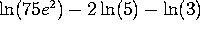Practice 2nd Midterm Exam -- Originally given November 3, 1992

Problem 1. Implicit differentiation

Consider the curve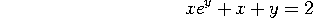a) Find dy/dx at the point (1,0).

b) Estimate the value of y when x=1.01. [Hint: use differentials and the result of part (a)]

Problem 2. Exponential Growth

According to the Encyclopedia Britannica, the population of Mexico was approximately 85,000,000 in 1990, and has been growing at 2.7% per year (compounded continuously). Assuming the population continues to grow at this rate,

a) What will the population be in the year 2050?

b) When will the population of Mexico hit 150,000,000?

Problem 3. Derivatives and graphing

Consider the function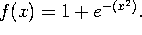a) Find the partition points (if any), the horizontal asymptotes (if any), and the vertical asymptotes (if any).

b) Find the critical points of f(x). For which values of x is f(x) an increasing function?

c) Find all the points of inflection of f(x). For what values of x is the graph of f(x) concave up?

d) Sketch the graph of f(x). Mark all the asymptotes (with A's), the partition points (with P's), the critical points (with C's), and the points of inflection (with I's).

Problem 4. Taking derivatives

Take the derivatives, with respect to x, of the following functions. Your final answer should be expressed entirely in terms of x. (e.g.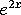, not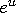). However, you do NOT need to simplify your results further.

a)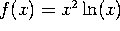b)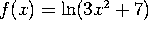c)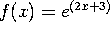d)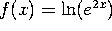Problem 5. Manipulating exponentials and logs

a) Convert this equation to log form: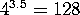b) Convert this equation to exponential form: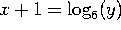c) Solve for x in the equation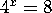d) Simplify as much as possible: